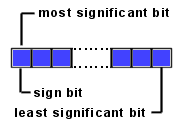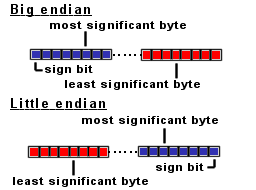# Java Quick Guide

This guide contains useful Java information.

## Java long type.

Information
Long types are so called signed two-complement numbers. The 2 complement scheme allows both positive and negative integers to be represented. The most significant bit of a two complement number is its sign bit. The sign bit is 1 for negative numbers and 0 for positive numbers and for 0.In Java the bytes are big-endian ordered, which means the most significant BYTE (where the sign bit is located) is always on the left side:The long value range is: 264 = 18446744073709551616

Half of this number (18446744073709551616) are positive numbers ranging from:
>=0 and <=9223372036854775807
For example:
0x0000 0x0000 0x0000 0x0000 = 0
0x0000 0x0000 0x0000 0x0001 = 1
0x7FFF 0xFFFF 0xFFFF 0xFFFF = 9223372036854775807

The other half are negative numbers ranging from:
>=-9223372036854775808 and <0
For example:
0xFFFF 0xFFFF 0xFFFF 0xFFFF = -1
0x8000 0x0000 0x0000 0x0000= -9223372036854775808

To convert negative decimal values into hexadecimal values:
value = 264 + (neg_dec_value)
value = 18446744073709551616 + (neg_dec_value)

For example:
neg_dec_value= -1
value = 18446744073709551616 + (neg_dec_value) = 18446744073709551616 + (-1) = 18446744073709551615
The new decimal value 18446744073709551615 equals 0xFFFF 0xFFFF 0xFFFF 0xFFFF (Use your Windows calculator, to verify this).

 Type Min value Max value Long -9223372036854775808 9223372036854775807